Test: Common Core: 6th Grade Math

 1 Call the three angles of a triangle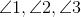.  The measure of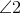is forty degrees less than that of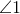; the measure of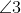is ten degrees less than twice that of. Ifis the measure of, then which of the following equations would we need to solve in order to calculate the measures of the angles?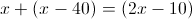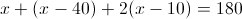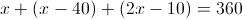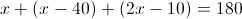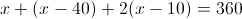1/5 questions

0%

Access results and powerful study features!

Take 15 seconds to create an account.